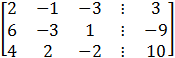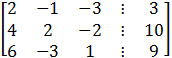### Sample ProblemSolve this system using Gaussian Elimination with back-substitution.

x=

y=

z=

#### Solution

Write the augmented matrix for the system.Switch E1withE3Switch E2 with E3E2=E2-2E1

E3=E3-3E1E1=E1/2

E2=E2/4

3=E3/10The matrix is now in row-echelon form, and the corresponding system isUsing back substitution (z=0), we can determine that

y+z=1 ,  y=1-z

y=1-0=1

Using back substitution again (z=0 and y=1), we can determine that

x-1/2y-3/2z=3/2

x=3/2+1/2y+3/2z

x=3/2+1/2(1)+3/2(0)=3/2+1/2=2